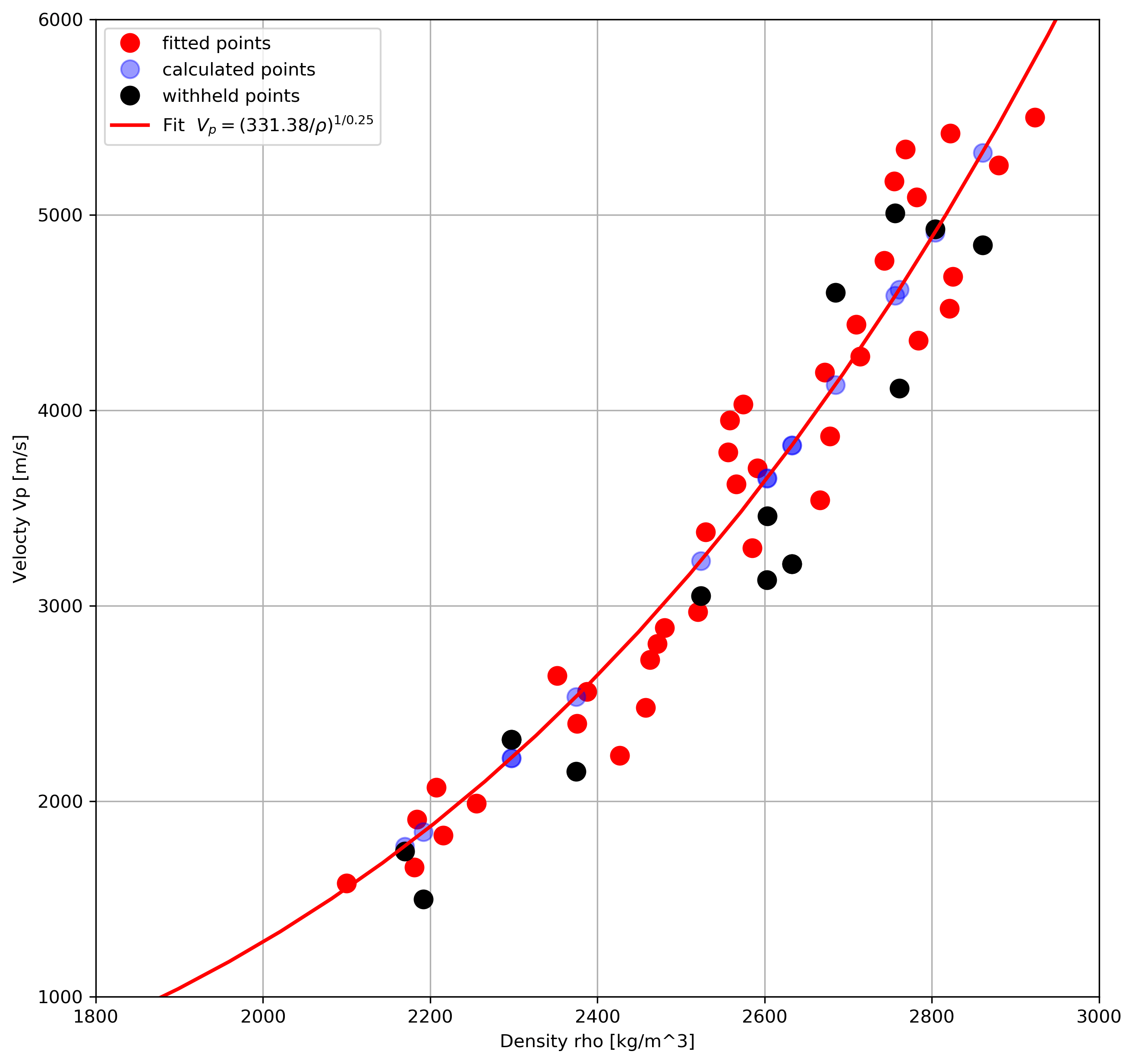# Geophysics Python sprint 2018 – day 2 and beyond, part I

In my last post I wrote about what I did on day one of the Geophysics sprint run by Agile Scientific in Santa Ana two weeks ago.

This post and the next one are about the project Volodymyr and I worked on during day two of the sprint, and continued to work on upon our return to Calgary.

We had read a great notebook by Alessandro Amato del Monte (I recommend browsing his Geophysical notes repo) showing how to reconstruct a velocity log from density with optimized alpha and beta parameters for the Inverse Gardner function, found via scipy.curve_fit.

Inspired by that, we set out with a dual goal:

• First, we wanted to adapt Alessandro’s optimization idea so that it would work with Bruges‘ Inverse Gardner
• Second, we wanted to adapt a function from some old work of mine to flag sections of the output velocity log with poor prediction; this would be useful to learn where alpha and beta may need to be tweaked because of changes in the rock lithology or fluid content

I’ll walk you through some of our work. Below are the two functions:

# Alessandro's simple inverse Gardner
def inv_gardner(rho, alpha, beta):
return (rho/alpha)**(1/beta)

# Bruges' inverse Gardner
def inverse_gardner(rho, alpha=310, beta=0.25, fps=False):
"""
Computes Gardner's density prediction from P-wave velocity.
Args:
rho (ndarray): Density in kg/m^3.
alpha (float): The factor, 310 for m/s and 230 for fps.
beta (float): The exponent, usually 0.25.
fps (bool): Set to true for FPS and the equation will use the typical
value for alpha. Overrides value for alpha, so if you want to use
your own alpha, regardless of units, set this to False.
Returns:
ndarray: Vp estimate in m/s.
"""
alpha = 230 if fps else alpha
exponent = 1 / beta
factor = 1 / alpha**exponent
return factor * rho**exponent

They look similarly structured, and take the same arguments. We can test them by passing a single density value and alpha/beta pair.

inv_gardner(2000, 0.39, 0.23)
>>> 1.349846231542594e+16

inverse_gardner(2000, 0.39, 0.23)
>>> 1.3498462315425942e+16

Good. So the next logical step would be to define some model density and velocity data (shamelessly taken from Alessandro’s notebook, except we now use Bruges’ Gardner with S.I. units) and pass the data, and Bruges’ inverse Gardner toscipy.curve_fit to see if it does just work; could it be that simple?

# Make up random velocity and density with Bruges' direct Gardner
vp_test = numpy.linspace(1500, 5500)
rho_test = gardner(vp_test, 310, 0.25)
noise = numpy.random.uniform(0.1, 0.3, vp_test.shape)*1000
rho_test = rho_test + noise

The next block is only slightly different from Alessandro’s notebook. Instead of using all data, we splits both density and velocity into two pairs of arrays: a rho12 and vp2 to optimize foralpha and beta,  a rho1 for calculating “unknown” velocities vp_calc1 further down; the last one, v1, will be used just to show where the real data might have been had we not had to calculate it.

idx = np.arange(len(vp_test))
np.random.seed(3)
spl1 = np.random.randint(0, len(vp_test), 15)
spl2 = np.setxor1d(idx,spl1)
rho1 = rho_test[spl1]
rho2 = rho_test[spl2]
vp1= vp_test[spl1] # this we pretend we do not have
vp2= vp_test[spl2]

Now, as in Alessandro’s notebook, we pass simple inverse Gardner function to scipy.curve_fit to find optimal alpha and beta parameters, and we printalpha and beta.

popt_synt2, pcov2 = scipy.curve_fit(inv_gardner,rho2, vp2)
print (popt_synt2)
>>> [3.31376056e+02 2.51257203e-01]

Those values seem reasonable, but just to be sure let’s calculate vp_calc1 from rho1 and plot everything to be sure.

vp_calc1 = inv_gardner(rho1, *popt_synt2)

# this is to show the fit line
rho_synt_fit=np.linspace(1, 3000, 50)
vp_synt_fit=inv_gardner(rho_synt_fit, *popt_synt2)

plt.figure(figsize=(10, 10))
plt.plot(rho2,vp2,'or', markersize = 10, label = "fitted points")
plt.plot(rho1,vp1,'ob', markersize = 10, alpha = 0.4, label = "calculated points")
plt.plot(rho1,vp1,'ok', markersize = 10, label = "withheld points")
plt.plot(rho_synt_fit, vp_synt_fit, '-r', lw=2,
label='Fit' r'$V_p=(%.2f / \rho)^{1/%.2f}$' %(popt_synt2,
popt_synt2))
plt.xlabel('Density rho [kg/m^3]'), plt.xlim(1800, 3000)
plt.ylabel('Velocty Vp [m/s]'), plt.ylim(1000, 6000)
plt.grid()
plt.legend(loc='upper left')
plt.show()That looks great. Let’s now try the same using Bruges’ Inverse Gardner.

popt_synt2, pcov2 = curve_fit(inverse_gardner, rho2, vp2)
print (popt_synt2)
>>> [1.         0.29525991 1.        ]

That is odd, we do not get the same parameters; additionally, there’s this error message:

../scipy/optimize/minpack.py:794:
OptimizeWarning: Covariance of the parameters could not be estimated
category=OptimizeWarning)

One possible explanation is that although both inv_gardner and inverse_gardner take three parameters, perhaps scipy.curve_fit does not know to expect it because in the latter alpha and betaare pre-assigned.

The workaround for this was to write a wrapper function to ‘map’ between the call signature of scipy.curve_fit and that of inverse_gardner so that it would be ‘communicated’ to the former explicitly.

def optimize_inverse_gardner(rho, alpha, beta):
return inverse_gardner(rho, alpha=alpha, beta=beta)

popt_synt2, pcov2 = scipy.curve_fit(optimize_inverse_gardner,
rho2, vp2)
print (popt_synt2)
>>> [3.31376060e+02 2.51257202e-01]

Which is the result we wanted.

In the next post we will apply this to some real data and show how to flag areas of poorer results.